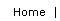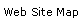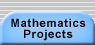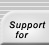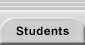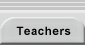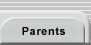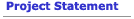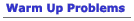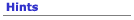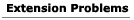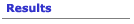## Extensions for the Simplex Lock Problem

#### 1 Any Number of Buttons

One way to extend the Simplex Lock Problem is to consider not just a 5-button lock, but locks with any number of buttons. If you have an n-button Simplex Lock, how many combinations will it have?

#### 2 Picking a Lock

The 5-button Simplex Lock has only 1,082 possible combinations. By comparison, this 3-dial lock (three wheels, each with digits 0-9) has 10 × 10 × 10 = 1, 000 possible combinations.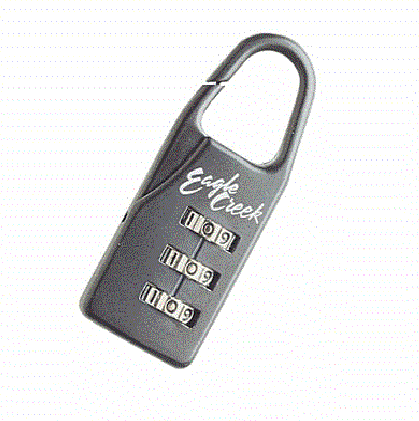The total number of combinations is not very different, but the Simplex Lock is much harder to pick because it is harder to systematically test each possible combination.

In the lock above, you can simply list numbers 000-999, lowest to highest, and you will test each combination. If you change the lock to 4 dials, the number of combinations is now 10,000, but you can still step through all of them using the same strategy: List the numbers 0000-9999.

How could you systematically test each combination of a 5-button Simplex Lock? This requires finding a way to list them all without missing any and without duplication. Can you extend your algorithm for lisiting combinations to an n-button lock?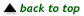#### 3 Stirling Numbers

A student in Oakland, California, came up with the following idea: You make a picture like Pascal’s triangle, except that in adding entries from one row to the next, each number on row N has a weight, which is its position within the row (starting from 1). For example: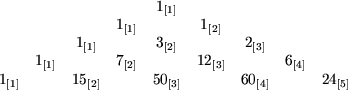The numbers in brackets are the weights. So for example the 7 in the fourth row is 1  ×  1 + 3  × 2. The 50 in the fifth row is 7 × 2 + 12 × 3. And the sum of each row is (starting at n = 0) is the number of combinations in an n-button Simplex lock.”

The numbers in this triangle are related to the famous “Stirling numbers of the second kind”. Find out about Stirling numbers and use them to find a solution to the Simplex lock problem.Translations of mathematical formulas for web display were created by tex4ht. © Copyright 2003 Education Development Center, Inc. (EDC)